# FileInputStream skip() Method in Java with Examples

• Last Updated : 01 Dec, 2021

FileInputStream class is quite helpful to read data from a file in the form of a sequence of bytes. FileInputStream is meant for reading streams of raw bytes such as image, audio, video, etc. For reading streams of characters, FileReader is recommended. It was firstly introduced in JDK 1.0.

### FileInputStream skip() method

The skip(n) method in FileInputStream is quite helpful as it discards the n bytes of data from the beginning of the input stream. We can understand its working by considering the following example,

We are reading a stream of characters through FileInputStream, and we want to read it after skipping the first eight characters from the stream. Let the string be “GeeksforGeeks“, so after skipping the first eight characters, our string will become “Geeks“.

The skip() method is useful to achieve the task as mentioned above. This method is defined in FileInputStream class of the Java.io package.

Java I/O package helps the user to perform all the input-output operations. These streams support all objects, data types, characters, files, etc., to execute the I/O operations fully.

Syntax:

```Input_File_Stream.skip(n)
Input_File_Stream: The file input stream.```

Parameters:

• n − A Non-negative integer.
• Bytes to be skipped from a stream of characters.

Return Value: It returns the actual number of bytes skipped.

Exception: IOException − If n is a negative integer or I/O error occurs.

## Java

 `// Java program to demonstrate the working``// of skip() method in FileInputStream`` ` `// Importing the class files``// defined under io Package``import` `java.io.FileInputStream;``import` `java.io.IOException;`` ` `// Public class``public` `class` `GeeksforGeeks {`` ` `    ``// Main method``    ``public` `static` `void` `main(String[] args)``        ``throws` `IOException``    ``{`` ` `        ``FileInputStream inputFileStream = ``null``;`` ` `        ``// Variables to store the fifth character``        ``int` `integerValue = ``0``;``        ``char` `characterValue;``        ``try` `{``            ``// Create new file input stream``            ``inputFileStream = ``new` `FileInputStream(``                ``"C:\\Users\\harsh\\Desktop\\GFG\\inputFile.txt"``);`` ` `            ``// Skip 4 bytes from the beginning``            ``// in the file input stream``            ``inputFileStream.skip(``4``);`` ` `            ``// Read bytes from this stream``            ``// (first character only)``            ``integerValue = inputFileStream.read();`` ` `            ``// Converting integer to character type``            ``characterValue = (``char``)i;`` ` `            ``// Print the character``            ``System.out.print(``"Character read: "``                             ``+ characterValue);``        ``}``        ``catch` `(Exception exception) {`` ` `            ``// If any error occurs``            ``exception.printStackTrace();``        ``}``        ``finally` `{`` ` `            ``// Releasing all system resources``            ``if` `(inputFileStream != ``null``)``                ``inputFileStream.close();``        ``}``    ``}``}``// This code is contributed by Bhuwanesh`

### Steps for Compilation in a Local System:

1. We have saved the above program with the name “GeeksforGeeks” locally: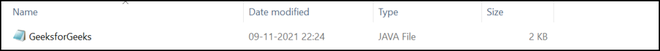Source code file

2. Lets now create a text file named “inputFile“: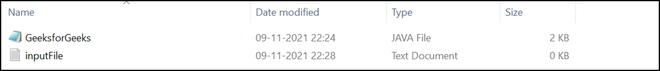Text file

3. We need to enter some text inside the created text file. As an example, we have written “Geeks

eeks” in the text file.Text file data

4. We will now compile our program through the javac compiler using the command prompt:

`javac GeeksforGeeks.java`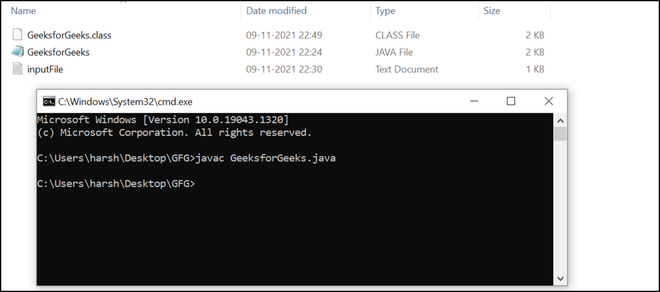Compilation of the source code file

5. As you can see below GeeksforGeeks.class byte code file is generated. Now run the program using the following command:

`java GeeksforGeeks`

Output: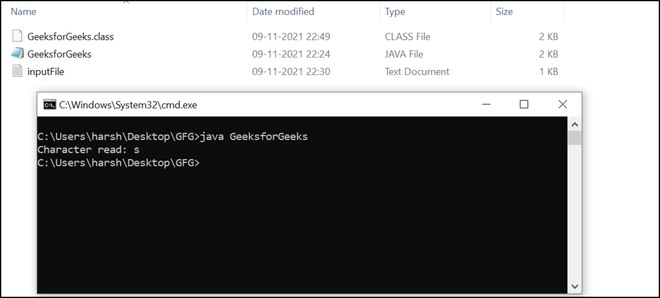Output

Output Description: Since we have passed 4 as an argument to the skip() function in the program. As we can see in the program’s output, the first four characters (or bytes) are skipped (‘G‘, ‘e‘, ‘e‘, ‘k‘ ). The fifth character in GeekforGeeks is ‘s‘ hence, ‘s‘ is printed on the console.

## Java

 `// Java program to demonstrate the working``// of skip() method in FileInputStream`` ` `// Importing the FileInputStream class``import` `java.io.FileInputStream;``// Importing the IOException class``import` `java.io.IOException;`` ` `// Public class``public` `class` `GeeksforGeeks {`` ` `    ``// Main method``    ``public` `static` `void` `main(String[] args)``        ``throws` `IOException``    ``{`` ` `        ``FileInputStream inputFileStream = ``null``;``        ``int` `integerValue = ``0``;``        ``char` `characterValue;``        ``try` `{``            ``// Create new file input stream``            ``// Give the full path of the input file``            ``inputFileStream = ``new` `FileInputStream(``                ``"C:\\Users\\harsh\\Desktop\\GFG\\inputFile.txt"``);`` ` `            ``// Skip 8 bytes from the beginning in the file``            ``// input stream``            ``inputFileStream.skip(``8``);`` ` `            ``// Print on console``            ``System.out.print(``"String read: "``);`` ` `            ``// Iterate till EOF is not reached``            ``while` `((integerValue = inputFileStream.read())``                   ``!= -``1``) {`` ` `                ``// converts integer to character``                ``characterValue = (``char``)i;`` ` `                ``// prints character``                ``System.out.print(characterValue);``            ``}``        ``}``        ``catch` `(Exception exception) {`` ` `            ``// If any error occurs``            ``exception.printStackTrace();``        ``}``        ``finally` `{`` ` `            ``// Releasing all system resources``            ``if` `(inputFileStream != ``null``)``                ``inputFileStream.close();``        ``}``    ``}``}``// This code is contributed by Bhuwanesh`

In order to compile the above program in a local system, We can follow the same steps that we have mentioned in Example 1. After the compilation, we can run the program, and it generates the following output.

Output: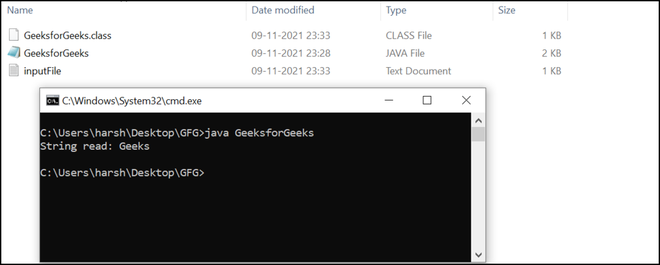Output

Output Description: As you can see in the output first eight characters are skipped (‘G‘, ‘e‘, ‘e‘, ‘k‘, ‘s‘, ‘f‘, ‘o‘, ‘r‘). The remaining string is “Geeks” so it gets printed on the console.

My Personal Notes arrow_drop_up